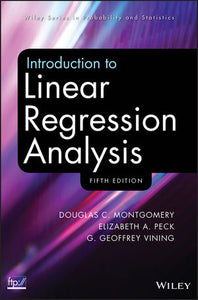# Introduction to Linear Regression Analysis, 5th Edition

Douglas C. Montgomery, Elizabeth A. Peck, G. Geoffrey Vining

Format: Print Book

ISBN: 9780470542811

• SGD 98.60
Unit price per
• Save SGD 134.85
Tax included.

A comprehensive and up-to-date introduction to the fundamentals of regression analysis Introduction to Linear Regression Analysis, Fifth Edition continues to present both the conventional and less common uses of linear regression in today’s cutting-edge scientific research. The authors blend both theory and application to equip readers with an understanding of the basic principles needed to apply regression model-building techniques in various fields of study, including engineering, management, and the health sciences. Following a general introduction to regression modeling, including typical applications, a host of technical tools are outlined such as basic inference procedures, introductory aspects of model adequacy checking, and polynomial regression models and their variations. The book then discusses how transformations and weighted least squares can be used to resolve problems of model inadequacy and also how to deal with influential observations. The Fifth Edition features numerous newly added topics, including: A chapter on regression analysis of time series data that presents the Durbin-Watson test and other techniques for detecting autocorrelation as well as parameter estimation in time series regression models Regression models with random effects in addition to a discussion on subsampling and the importance of the mixed model Tests on individual regression coefficients and subsets of coefficients Examples of current uses of simple linear regression models and the use of multiple regression models for understanding patient satisfaction data. In addition to Minitab, SAS, and S-PLUS, the authors have incorporated JMP and the freely available R software to illustrate the discussed techniques and procedures in this new edition. Numerous exercises have been added throughout, allowing readers to test their understanding of the material. Introduction to Linear Regression Analysis, Fifth Edition is an excellent book for statistics and engineering courses on regression at the upper-undergraduate and graduate levels. The book also serves as a valuable, robust resource for professionals in the fields of engineering, life and biological sciences, and the social sciences.

Imprint: Wiley
Language: English
Publication Year: 2012
Format: Hardcover
Course Code:MTH357,MTH358,SUSS